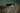Recap of Year 12 VCE Chemistry: Part 2Step 1
>
Step 2
>
Step 3
>
Step 4
How would you like to be tutored?
Online
In Person

# Recap of Year 12 VCE Chemistry: Part 2

This article will focus on the key concepts that will be covered in Unit 4 VCE Chemistry.

Unit 4 is concerned mainly about industrial chemistry that is the industrial production of chemicals and the energy changes that accompanies chemical reactions.

In this unit you will also look at the different sources of energy that are available to use as well as the chemical reactions that take place in order to produce that energy that we use.

Unit 4 consists of two areas of studies:

• Area of Study 1: Industrial Chemistry
• Area of Study 2: Supplying and using energy

Area of Study 1- Industrial Chemistry:

This area of study will focus on factors that affect the rate and extent of a chemical reaction. When chemicals are produced in the industry, a compromise needs to be reached between rate and yield.

In this area of study you should know:

• what the collision theory is and factors that affect the rate of a reaction namely, temperature, pressure, concentration and use of catalysts;
• how to draw energy profile diagrams as well as how to interpret them if you are given one in the exam;
• how the energy profile diagram will differ between catalysed reaction and non-catalysed reactions as well as between endothermic and exothermic reactions;
• what is ΔH and understand that it is specific for each type of reaction;
• how to deduce ΔH for an overall reaction given energy profiles;
• what is activation energy;
• what is an equilibrium reaction – a difference between a reversible and non-reversible reaction;
• what the equilibrium law;
• how to calculate the equilibrium constant (K value) for a reaction;
• how temperature affects the equilibrium of a reaction;
• Le Chatelier’s Principle ➔ you should know it off by heart by the end of this AOS;
• what factors affect the position of equilibrium. ➔ Also know how to interpret and draw the equilibrium graphs that may be present on an exam or SAC;
• how to calculate the pH ➔ What is the acidity constant and how do you use that to calculate the pH of a weak acid? What is the ionic product of water?
• the industrial chemical (ammonia, sulfuric acid or nitric acid ) that you will learn about in your class. (NOTE: Each school will pick one of these chemicals to study in detail, it will be assessed in one of your Unit 4 SACs, but will not be examined specifically in the exam.)

Area of Study 2- Supplying and using energy:

This AOS will focus on the use of different energy resources- both renewable and non-renewable sources. You will also look at how we can measure the energy of a chemical reaction.

It is in this AOS that the electrochemical series in your data book will be put into use.

In this AOS you should know:

• the similarities and differences, advantages and disadvantages of the following energy sources:
• coal
• petroleum
• natural gas
• nuclear fuels
• biochemical fuels
• know the reactions that the above energy sources undergo to produce energy;
• what a calorimeter is and what it is used for;
• the difference between a bomb calorimeter and solution calorimeter;
• how to perform the calculations to calibrate a calorimeter;
• what heat loss does to the calorimeter in terms of the ΔH value that is obtained;
• what is specific heat capacity;
• how to interpret the electrochemical series ➔ predicting redox reactions and deducing overall equations from the half-equations given;
• the limitations of the electrochemical series;
• how to calculate the maximum cell voltage;
• what galvanic cells are ➔ principles (anode, cathode, where oxidation/reduction occurs etc. …), half-equations and overall equations.
• Batteries (primary and secondary cells) and the reactions associated with them both for discharge and recharge stages;
• what fuel cells are ➔ principles, equations associated with the different types of fuel cells, advantages and disadvantages of fuel cells;
• what electrolytic cells are ➔ principles, equations associated with them, a comparison between electrolytic cells that use molten and aqueous electrolytes as well as inert and non-inert electrodes;
• what Faraday’s law are ➔ know the calculations that are associated with Faraday’s laws.

This is just an overview of what you will be encountering in Unit 4 Chemistry. The list is by no means exhaustive and there will be little things that you will learn in your classes that will not have been mentioned in this article.

Always refer back to the study design for a more detailed guide on what you should be learning and ask your teacher if you are ever unsure. Use this article as a supplement.

Disclaimer: This list represents what I think will be covered in Unit 4 Chemistry. The list is based on what is in the Study Design provided by VCAA. This article is in no way endorsed by VCAA and all views expressed here are my own and from my interpretation of the Study Design.